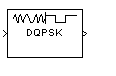DQPSK Demodulator Baseband

Demodulate DQPSK-modulated data

Library

PM, in Digital Baseband sublibrary of Modulation

•Description

The DQPSK Demodulator Baseband block demodulates a signal that was modulated using the differential quadrature phase shift keying method. The input is a baseband representation of the modulated signal.

The input must be a discrete-time complex signal. The output depends on the phase difference between the current symbol and the previous symbol. The first integer (or binary pair, if you set the Output type parameter to Bit) at the block output is the initial condition of zero because there is no previous symbol.

This block accepts either a scalar or column vector input signal. For information about the data types each block port supports, see Supported Data Types.

Outputs and Constellation Types

When you set Output type parameter to Integer, the block maps a phase difference of

θ + πm/2

to m, where θ represents the Phase rotation parameter and m is 0, 1, 2, or 3.

When you set the Output type parameter to Bit, then the output contains pairs of binary values. The reference page for the DQPSK Modulator Baseband block shows which phase differences map to each binary pair, for the cases when the Constellation ordering parameter is either Binary or Gray.

Dialog BoxOutput type

Determines whether the output consists of integers or pairs of bits.

Constellation ordering

Determines how the block maps each integer to a pair of output bits.

This phase difference between the current and previous modulated symbols results in an output of zero.

Output data type

When the parameter is set to 'Inherit via internal rule' (default setting), the block will inherit the output data type from the input port. The output data type will be the same as the input data type if the input is of type single or double.

For integer outputs, this block can output the data types int8, uint8, int16, uint16, int32, uint32, single, and double. For bit outputs, output can be int8, uint8, int16, uint16, int32, uint32, boolean, single, or double.

Supported Data Types

PortSupported Data Types

Input

• Double-precision floating point

• Single-precision floating point

Output

• Double-precision floating point

• Single-precision floating point

• Boolean when Output type isBit

• 8-, 16-, and 32-bit signed integers

• 8-, 16-, and 32-bit unsigned integers

Pair Block

DQPSK Modulator Baseband

Extended Capabilities

C/C++ Code GenerationGenerate C and C++ code using Simulink® Coder™.

Introduced before R2006a